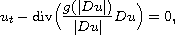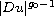Electron. J. Diff. Equ., Vol. 2015 (2015), No. 287, pp. 1-32.

### Holder continuity of bounded weak solutions to generalized parabolic p-Laplacian equations I: degenerate case Sukjung Hwang, Gary M. Lieberman

Abstract:
Here we generalize quasilinear parabolic p-Laplacian type equations to obtain the prototype equationwhere g is a nonnegative, increasing, and continuous function trapped in between two power functionsandwith. Through this generalization in the setting from Orlicz spaces, we provide a proof for the Holder continuity of such solutions which has much in common with that proof of Holder continuity of solutions of singular equations.

Submitted July 17, 2015. Published November 19, 2015.
Math Subject Classifications: 35B45, 35K65.
Key Words: Quasilinear parabolic equation; degenerate equation; generalized structure; a priori estimate; Holder continuity.

Show me the PDF file (399 KB), TEX file, and other files for this article.Sukjung Hwang Center for Mathematical Analysis and Computation Yonsei University, Seoul 03722, Korea email: sukjung_hwang@yonsei.ac.kr Gary M. Lieberman Department of Mathematics Iowa State University Ames, IA 50011, USA email: lieb@iastate.edu